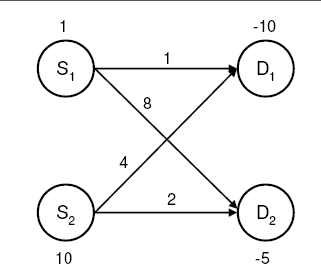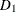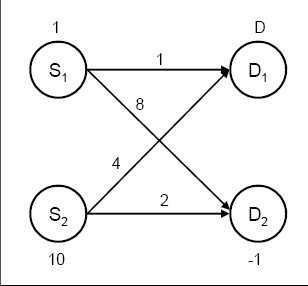### Example 6.9 Pure Networks: Using the EXCESS= Option

In this example we illustrate the use of the EXCESS= option for various scenarios in pure networks. Consider a simple network as shown in Output 6.9.1. The positive numbers on the nodes correspond to supply and the negative numbers correspond to demand. The numbers on the arcs indicate costs.

#### Transportation Problem, Total Supply < Total Demand

We first analyze a simple transportation problem where total demand exceeds total supply, as seen in Output 6.9.1. The EXCESS=SLACKS option is illustrated first.

Output 6.9.1: Transportation ProblemThe following SAS code creates the input data sets.

```data parcs;
input _from_ \$ _to_ \$ _cost_;
datalines;
s1 d1 1
s1 d2 8
s2 d1 4
s2 d2 2
;

data SleD;
input _node_ \$ _sd_;
datalines;
s1   1
s2  10
d1 -10
d2  -5
;
```

You can solve the problem using the following call to PROC NETFLOW:

```title1 'The NETFLOW Procedure';
proc netflow
excess   = slacks
arcdata  = parcs
nodedata = SleD
conout   = solex1;
run;
```

Since the EXCESS=SLACKS option is specified, the interior point method is used for optimization. Accordingly, the `CONOUT=` data set is specified. The optimal solution is displayed in Output 6.9.2.

Output 6.9.2: Supply < Demand

 The NETFLOW Procedure

Obs _from_ _to_ _cost_ _CAPAC_ _LO_ _SUPPLY_ _DEMAND_ _FLOW_ _FCOST_
1 s1 d1 1 99999999 0 1 10 1 1
2 s2 d1 4 99999999 0 10 10 5 20
3 s1 d2 8 99999999 0 1 5 0 0
4 s2 d2 2 99999999 0 10 5 5 10

The solution with the THRUNET option specified is displayed in Output 6.9.3.

```title1 'The NETFLOW Procedure';
proc netflow
thrunet
excess   = slacks
arcdata  = parcs
nodedata = SleD
conout   = solex1t;
run;
```

Output 6.9.3: Supply < Demand, THRUNET Specified

 The NETFLOW Procedure

Obs _from_ _to_ _cost_ _CAPAC_ _LO_ _SUPPLY_ _DEMAND_ _FLOW_ _FCOST_
1 s1 d1 1 99999999 0 1 10 5 5
2 s2 d1 4 99999999 0 10 10 5 20
3 s1 d2 8 99999999 0 1 5 0 0
4 s2 d2 2 99999999 0 10 5 5 10

Note: If you want to use the network simplex solver instead, you need to specify the EXCESS=ARCS option and, accordingly, the `ARCOUT=` data set.

#### Missing D Demand

As shown in Output 6.9.4, nodehas a missing D demand value.

Output 6.9.4: Missing D DemandThe following code creates the node data set:

```data node_missingD1;
input _node_ \$ _sd_;
missing D;
datalines;
s1   1
s2  10
d1   D
d2  -1
;
```

You can use the following call to PROC NETFLOW to solve the problem:

```title1 'The NETFLOW Procedure';
proc netflow
excess   = slacks
arcdata  = parcs
nodedata = node_missingD1
conout   = solex1b;
run;
```

The optimal solution is displayed in Output 6.9.5. As you can see, the flow balance at nodes with nonmissing `supdem` values is maintained. In other words, if a node has a nonmissing supply (demand) value, then the sum of flows out of (into) that node is equal to its `supdem` value.

Output 6.9.5: THRUNET Not Specified

 The NETFLOW Procedure

Obs _from_ _to_ _cost_ _CAPAC_ _LO_ _SUPPLY_ _DEMAND_ _FLOW_ _FCOST_
1 s1 d1 1 99999999 0 1 D 1 1
2 s2 d1 4 99999999 0 10 D 9 36
3 s1 d2 8 99999999 0 1 1 0 0
4 s2 d2 2 99999999 0 10 1 1 2

#### Missing D Demand, THRUNET Specified

Consider the previous example, but with the THRUNET option specified.

```title1 'The NETFLOW Procedure';
proc netflow
thrunet
excess   = slacks
arcdata  = parcs
nodedata = node_missingD1
conout   = solex1c;
run;
```

The optimal solution is displayed in Output 6.9.6. By specifying the THRUNET option, we have actually obtained the minimum-cost flow through the network, while maintaining flow balance at the nodes with nonmissing supply values.

Output 6.9.6: Missing D Demand, THRUNET Specified

 The NETFLOW Procedure

Obs _from_ _to_ _cost_ _CAPAC_ _LO_ _SUPPLY_ _DEMAND_ _FLOW_ _FCOST_
1 s1 d1 1 99999999 0 1 D 1 1
2 s2 d1 4 99999999 0 10 D 0 0
3 s1 d2 8 99999999 0 1 1 0 0
4 s2 d2 2 99999999 0 10 1 10 20

Note: The case with missing S supply values is similar to the case with missing D demand values.